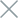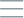• Home
• Tags
• Series
•# Python Standard Libraries: Math, Random, Datetime, OS

Python comes with a number of useful standard libraries to assist with common tasks in programming. Here is an introduction to popular standard libraries like `math`, `random`, `datetime` and `os`:

## `math` Library

The `math` library provides mathematical functions and operations. It allows you to perform complex calculations such as rounding numbers, computing logarithms, calculating factorials, and more.

Example:

``````import math

print(math.sqrt(25))   # Output: 5.0
print(math.factorial(5))   # Output: 120
``````

## `random` Library

The `random` library provides tools for working with random numbers. You can generate random numbers, choose a random element from a list, or perform various random-related tasks.

Example:

``````import random

print(random.random())   # Output: a random float between 0 and 1
print(random.randint(1, 10))   # Output: a random integer between 1 and 10
``````

## `datetime` Library

The `datetime` library offers tools for working with dates and times. It allows you to obtain the current date, format time, and calculate the difference between two dates.

Example:

``````import datetime

current_date = datetime.date.today()
print(current_date)   # Output: current date in the format 'YYYY-MM-DD'

current_time = datetime.datetime.now()
print(current_time)   # Output: current date and time in the format 'YYYY-MM-DD HH:MM:SS'
``````

## `os` Library

The `os` library provides tools for interacting with the operating system. You can perform tasks such as creating and deleting directories, getting a list of files in a directory, changing the current working directory, and more.

Example:

``````import os

current_dir = os.getcwd()
print(current_dir)   # Output: current working directory

os.mkdir("new_folder")   # create a new folder named "new_folder"
``````

These libraries in Python make it easy and efficient to perform common tasks. Additionally, Python has many other libraries to handle various tasks in programming.